# Projectile MotionPage 1

#### WATCH ALL SLIDES

Slide 1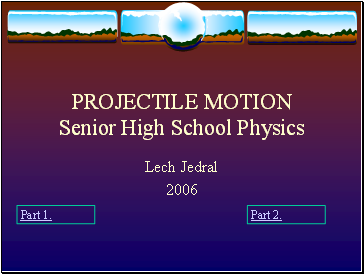PROJECTILE MOTION Senior High School Physics

Lech Jedral

Slide 2## Introduction

Projectile Motion:

Motion through the air without a propulsion

Examples:

Slide 3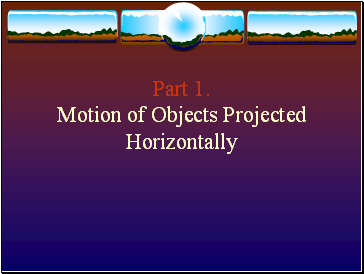Part 1. Motion of Objects Projected Horizontally

Slide 4v0

x

y

Slide 5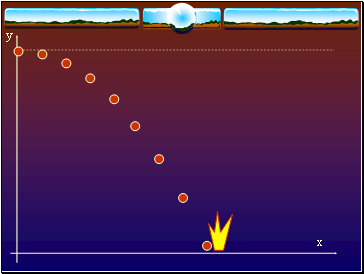x

y

Slide 6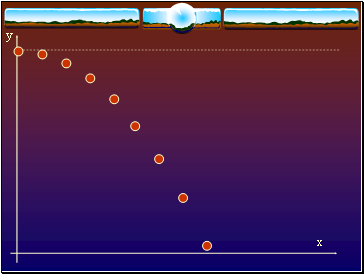x

y

Slide 7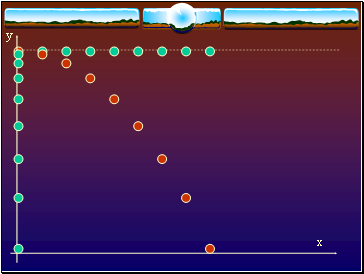x

y

Slide 8x

y

Slide 9x

y

Motion is accelerated

Acceleration is constant, and downward

a = g = -9.81m/s2

The horizontal (x) component of velocity is constant

The horizontal and vertical motions are independent of each other, but they have a common time

g = -9.81m/s2

Slide 10## Analysis of motion

ASSUMPTIONS:

x-direction (horizontal): uniform motion

y-direction (vertical): accelerated motion

no air resistance

QUESTIONS:

What is the trajectory?

What is the total time of the motion?

What is the horizontal range?

What is the final velocity?

Slide 11Frame of reference:

Equations of motion:

Slide 12## Trajectory

x = v0 t

y = h + ½ g t2

Eliminate time, t

t = x/v0

y = h + ½ g (x/v0)2

y = h + ½ (g/v02) x2

y = ½ (g/v02) x2 + h

Parabola, open down

Slide 13Total Time, Δt

y = h + ½ g t2

final y = 0

ti =0

tf =Δt

0 = h + ½ g (Δt)2

Solve for Δt:

Total time of motion depends only on the initial height, h

Δt = tf - ti

Slide 14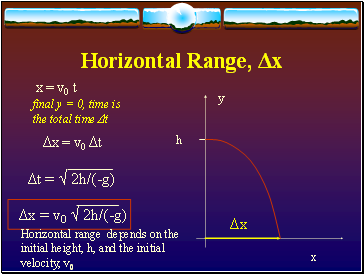Horizontal Range, Δx

final y = 0, time is the total time Δt

Horizontal range depends on the initial height, h, and the initial velocity, v0

x = v0 t

Δx = v0 Δt

Go to page:
1  2  3